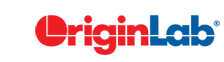# 17.8.8 (PSS) Two-Variance Test

Use this feature to determine the power of a two-sample variance test given the sample size or the sample size required to obtain a specified power for two-sample variance test .

Generally speaking, Power and Sample Size tools can be used in two ways: sample-size estimation and power computation. The former can be used to determine the size of the sample to ensure a certain power when you are designing an experiment. The latter can be used to estimate the accuracy of the result after you have conducted an experiment on a specific number of samples.

To perform a Power and Sample Size for two-sample variance test :

1. Select Statistics: Power and Sample Size: (PSS) Two-Variance Test. This menu command opens the dialog box.
2. From the Calculate list, choose Power or Sample Size, then choose your remaining options.
3. Upon clicking OK, an analysis report sheet is generated.
 Topics covered in this section: